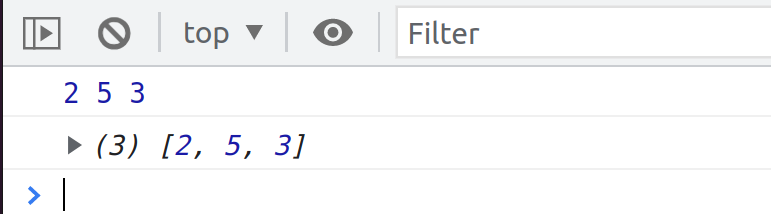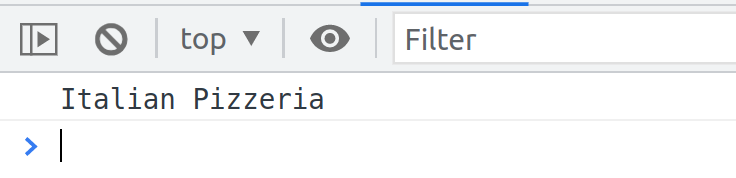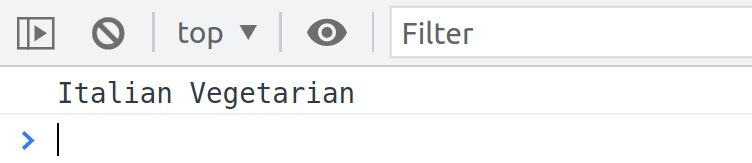# const - JS | lectureData Structures, Modern Operators & Strings

Destructuring Arrays

Let's start this section by learning about array destructuring. In this new section, we're going to continue learning basic syntax and basic JavaScript features, but now with a focus on more modern JavaScript.

The theme of this section will be to simulate a food delivery application for an Italian restaurant called "Epoca." Below is the starter data.

script.js
``````const restaurant = {
name: 'Epoca',
location: '17 Rue Oudinot, 75007 Paris',
categories: ['Italian', 'Pizzeria', 'Vegetarian', 'Organic'],
};``````

Now learning complex topics like the ones we're going to dive into in this section is best done in isolation. This way, you can understand exactly what I'm trying to teach you without thinking about five other things at the same time. Hence, I'm isolating just this one restaurant and building no user interface so that we can focus just on the JavaScript language here.

So destructuring is an ES6 feature, and it's a way of unpacking values from an array or an object into separate variables. In other words destructuring is to break a complex data structure down into a smaller data structure like a variable.

For arrays, we use destructuring to retrieve elements from the array and store them into variables very easily.

script.js
``const arr = [2, 5, 3];``

Now, if we wanted to retrieve each one into its own variable without destructuring, we would do it like this.

script.js
``````const a = arr;
const b = arr;
const c = arr;``````

But now, with destructuring, we can declare all the three variables at the same time.

script.js
``````const [x, y, z] = arr;
console.log(x, y, z);``````From the console, you can see that we have 2, 5, and 3, which are the values of x, y, and z, respectively. So doing `[x, y z]` on the left side of the equal operator destructures or is destructuring the array `arr` on the right side.

`[x,y,z]` might look like an array, but it's not. It's just the destructuring assignment. So whenever JavaScript sees `[x,y,z]` on the left side of the equal sign, it knows that it should do destructuring.

When destructuring, don't forget to declare the variables using `const`. I also wanted to note that the original array is not affected even though we did destructuring.

script.js
``````const [x, y, z] = arr;
console.log(x, y, z);
console.log(arr)``````We are not destroying the array arr. We are only destructuring or unpacking it.

Now let's work with the data from our `restaurant`. We can see that we have a couple of arrays: categories, starterMenu, and mainMenu.

Let's take some elements out of the `categories`.

script.js
``const [first, second] = restaurant.categories;``

You can see that we do not have to take all of the elements out of the array. The above will just take the first and the second element. It will simply follow the order of the elements in the `categories` array.

Let's now check their values in the console

script.js
``console.log(first, second);``We get Italian and Pizzeria, which are exactly the first two elements of the array. Now, let's say that we only wanted to take the first and third categories. That is, Italian and Vegetarian.

To do that, we leave a hole in the destructuring operator.

script.js
``const [first, , third] = restaurant.categories;``

Doing this will now make the second element to be skipped.We can now see that `third` is Vegetarian, which is element number three. By doing this, we don't have to create variables for stuff that we might not even need. So if we only need to take Italian and Vegetarian, we just skip the element in the middle.

This is really powerful, and we use destructuring to do many cool things. For example, let's say that the restaurant owner now decided to switch the first and the third category.

So right now, the first is Italian, and the third is Vegetarian. But now they want to switch it. Without destructuring, we would have to do this using a temporary variable.

script.js
``````const temp = first;first = third;third = temp;
console.log(first, third);``````Now with destructuring, we can make it a lot easier.

script.js
``[first, third] = [third, first]``

We're not using `let` or `const` here because we are simply reassigning the values of the two variables. And also, we do not need a temporary variable.

Let's check the result in the console.We can see that the result is the same, but this is a lot easier, and it is a trick that I use all the time. Another trick with destructuring is that we can have a function, return an array, and immediately destruct the result into different variables. This basically allows us to return multiple values from a function.

Let's try that and write a function to order food.

Let's start by adding a method in our `restaurant` object called `order`, which takes two parameters: an index for the starter menu and an index for the main menu.

script.js
``````const restaurant = {
// PROPERTIES

Right now, we are not doing any destructuring yet, but let's now call the method and destructure the returned array.

script.js
``````console.log(restaurant.order(1, 0));
const [starter, main] = restaurant.order(1, 0);
console.log(starter, main);``````You can see that we get the Bruschetta and Pizza in the console. This is how we basically receive two returned values from a function. Of course, we could have done that without destructuring and still returning an array from the function, but this is a very handy way to create two variables out of one function call immediately.

Now that you understand how destructuring works let's take it one step further because what happens if we have a nested array?

script.js
``const nestedArr = [3, 5, [8, 2]];``

As a small exercise, how can we get the value `3` and the array `[8,2]`? Well, we define two new variables using the destructuring assignment.

script.js
``````const [i, , j] = nestedArr;
console.log(i, j);``````We get the number 3 and then the array eight and two. That's, but what if we wanted all the individual values? Well, then we would essentially have to do destructuring inside of destructuring. That seems complicated, but it's not.

script.js
``````const [i, , [j, k]] = nestedArr;
console.log(i, j, k);``````And voila! So that's how nested destructuring works. Basically, we need to do destructuring inside of destructuring.

To finish, there is just another feature of destructuring that I want to show you. We can also set default values for the variables when extracting them. That's going to be very useful if we don't know the length of the array, and this can sometimes happen in real-world applications, as you will see later.

So if we have an array shorter than we might think, then we might try to unpack the array in positions that don't even exist.

script.js
``````// Let's just pretend that we don't know this array
const unknownArr = [7, 4];
const [p, q, r] = unknownArr;``````

When we then try to log them

``console.log(p, q, r);``You see that we get `undefined` as the third one. So this would basically be the same as trying to read the element at position number two (`unknownArr`) of this array, but it only has elements at positions zero and one. Hence, therefore we then get `undefined`.

But as I said, we can set default values. So let's simply set them all to one and then, our `r` will become one.

script.js
``````const [p = 1, q = 1, r = 1] = unknownArr;
console.log(p, q, r);``````And if we remove the second element in the array, then `q` will also become one. And as I said, this can sometimes be useful. For example, when we get data from an API. And so we're going to probably use this one and actually everything that we did in his lecture a bit later in the course.